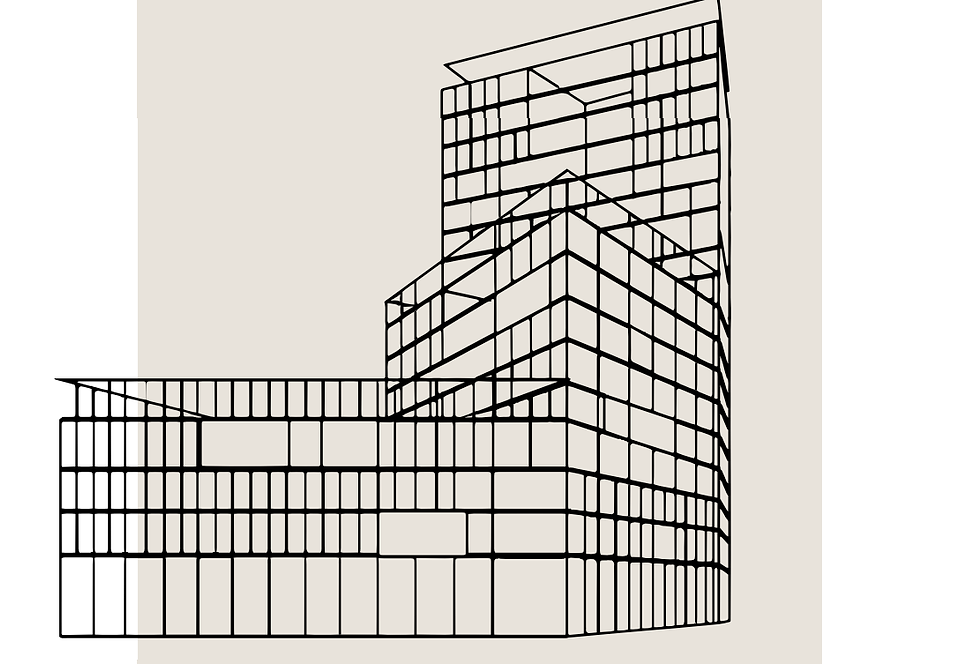top of page
Search

# What is a Fibonacci?

Fibonacci is being built on the corner of Panamalaan and Zeeburgerdijk in Amsterdam East. A residential building with communal facilities for 194 young people. But what exactly is Fibonacci?Fibonacci was an Italian mathematician. His real name was Leonardo of Pisa. The word Fibonacci refers to a series that he published in his book “Lber Abaci” around the year 1202. This series looks like this: 0,0.1,1,2,3,5,8,13,21..etc The series shows a growth pattern that also occurs in nature.Namely, each number is created by adding the previous two together.

Just look: 3+5 = 8 and 8+13 = 21.

If you draw these numbers in boxes: so number 1 as a square of 1:1 cm, number 2 as a square of 2:2, etc., you get a pattern.If you connect the vertices of all these squares together, you get a smooth shape that occurs a lot in nature;like the galaxy, a snail shell you name it.

Can you already discover the Fibonacci sequence on the residential building?